## Final exam

The final exam took place on Thursday August 8, at 8:30 AM in the usual lecture Hall E. The Exam scheduleof summer school.
Exam
Exam Solutions
Some practice exams: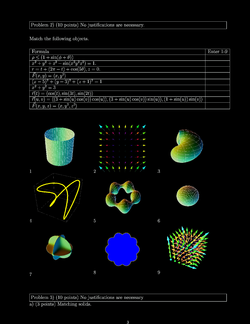Practice I, [Solutions]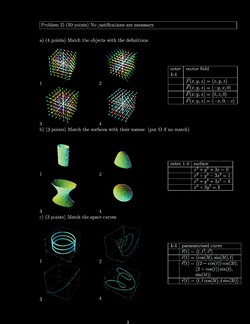Practice II, [Solutions]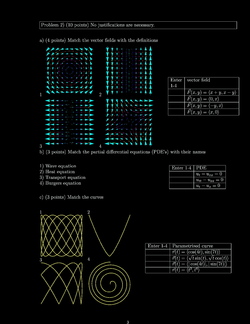Practice III, [Solutions]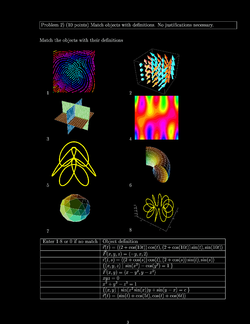Practice IV, [Solutions]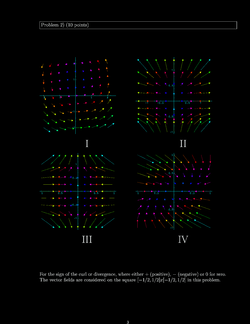Practice V, [Solutions]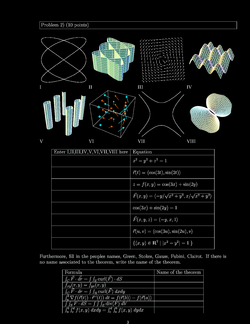Practice VI, [Solutions]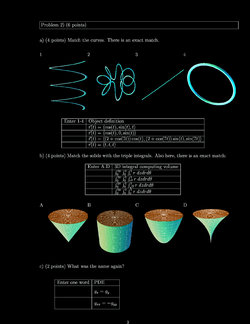Practice VII, [Solutions]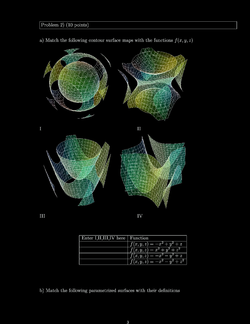Practice VIII, [Solutions]

## Second hourly

The second hourly covered the material from week 3 and 4 and took place on Thursday July 25 2013, in the usual lecture Hall E.

Exam
Exam Solutions
Here are old practice exams: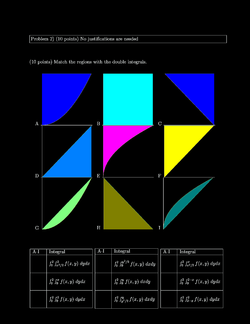Practice I, [Solutions]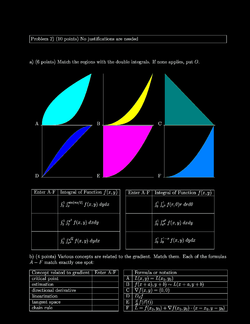Practice II, [Solutions]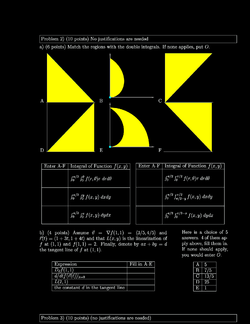Practice III, [Solutions]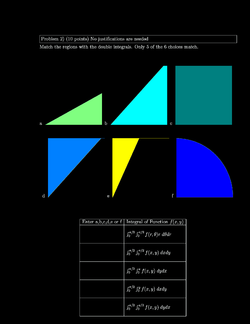Practice IV, [Solutions]

Just in case, you need more practice for the other type of problems, here are some more: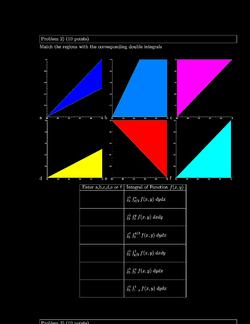Practice V, [Solutions]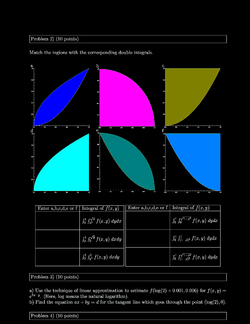Practice VI, [Solutions]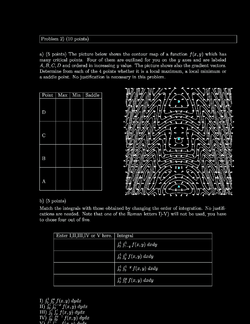Practice VII,[Solutions]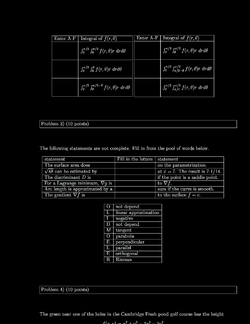Practice VIII, [Solutions]

## First hourly

The first hourly covered all the material from the first two weeks and the definition of the partial derivative. We had a review on Tuesday, July 9. The exam took place on July 11. Here are some practice exams: (there are way too many, but look at one of them!)

Exam
Exam Solutions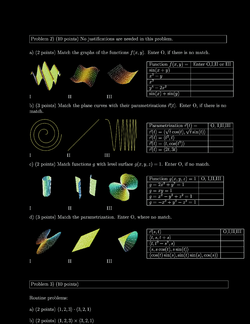Practice I, [Solutions]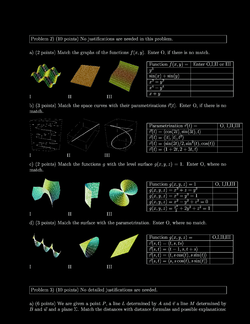Practice II, [Solutions]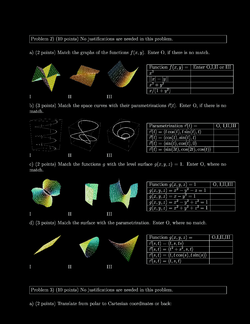Practice III, [Solutions]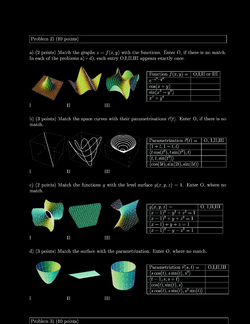Practice IV, [Solutions]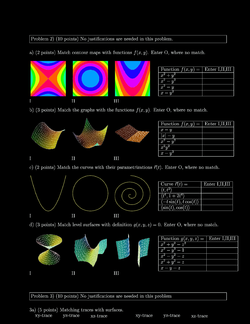Practice V, [Solutions]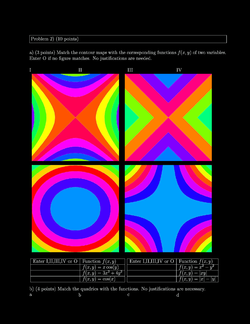Practice VI, [Solutions]